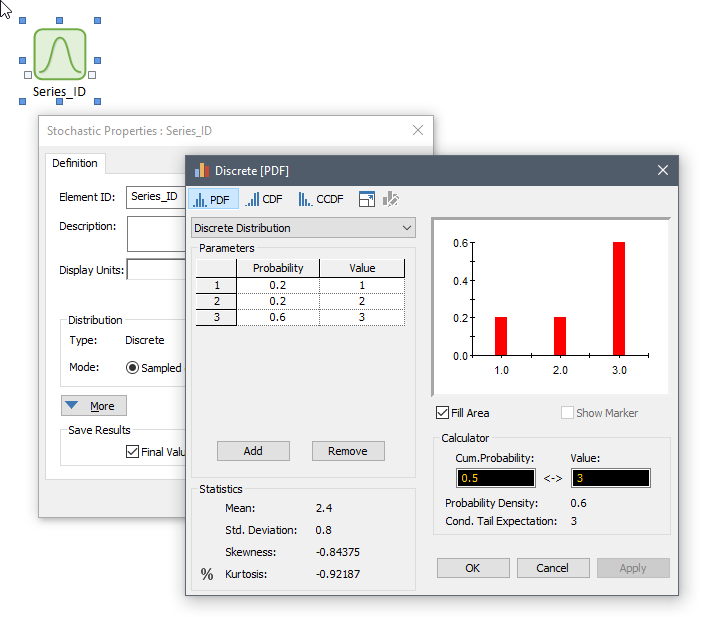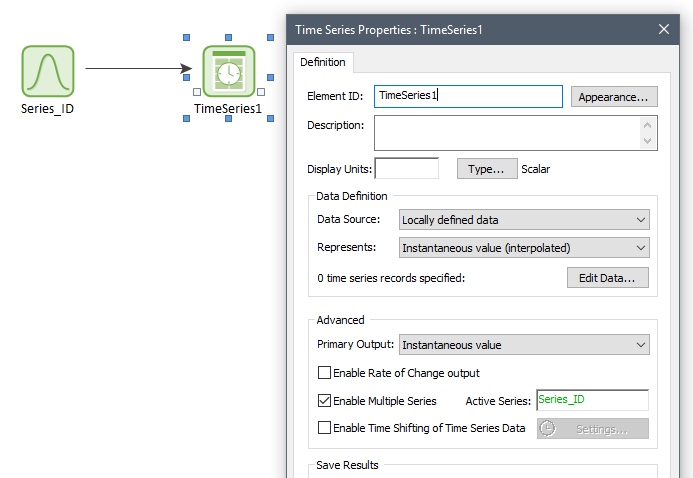•Jason

Ebrahim,

When you say "assign a probability" to each series, do you mean that you want to define the probability that a particular series is selected? So in the example you present, there is a 20% chance that the first series is selected and so on? By the way, if this is the case, then the probabilities would need to add up to 1.0.

•Yes. That's exactly what I meant. Thanks Jason!

But where/how do we input those probabilities in GoldSim? I can define multiple time series but don't know how to assign a unique probability to each.

•Jason

This is how I might do something like this:

Set up a Stochastic element with a discrete probability called "Series_ID" that will be used to select the time series.Next, refer to Series_ID in your time series multiple series input definition.••